# Reducing Testing Time of Pharmaceutical Drugs

The ‘shelf life’ is the total amount of time that a product can be stored and still be both safe and effective for use. Pharmaceutical shelf life can be affected by a number of factors, such as:

• Light
• Temperature conditions
• Humidity
• Gas atmosphere

Determining the shelf life of a product usually requires time consuming tests.

How can the shelf life of drugs be tested, without requiring long testing times? Early predictions about the shelf life of a drug, in particular its thermal stability, can be achieved using thermogravimetric measurements with kinetics evaluation. This can take between hours and days to carry out.

## What is Required to Obtain 5-year Forecasts in a Few Hours?

• A thermobalance, a device that stores the mass variations of the sample during its heating
• Kinetics Neo, a software that determines the kinetics of the decomposition reaction

## What are the Next Steps?

1. Take TGA measurements at different heating rates
2. Undertake the kinetics evaluation with the Kinetics Neo software
3. Predict the sample behavior for specified temperatures and times using the kinetics model
4. Compare a measurement at an isothermal temperature with the curve calculated by Kinetics Neo to validate the kinetic model

As an example, potassium clavulanate will be used. This is a pharmaceutical substance which is usually used with the antibiotic amoxicillin in order to increase its effectiveness.

1. TG measurements at different heating rates

Figure 1 displays the TGA and DTG (first derivative) curves of the measurement on potassium clavulanate at 10 K/min in a dynamic nitrogen atmosphere. The initial mass-loss step is identified between room temperature and 120 °C.

This is caused by surface water evaporating. After this there are three more mass-loss steps which are found between 120 °C and 600 °C, these are due to the decomposition of potassium clavulanate.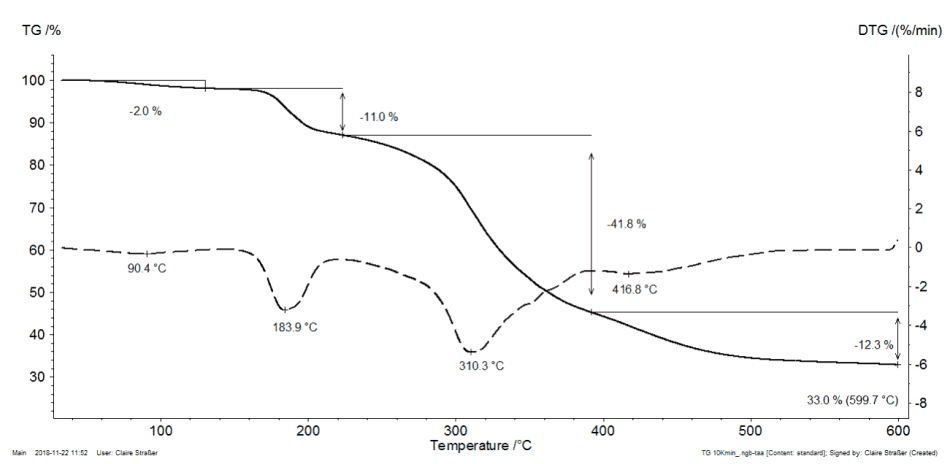Figure 1. TGA measurement on potassium clavulanate in pierced crucibles at 10 K/min in a dynamic nitrogen atmosphere, solid lines: TGA, dashed lines: DTG.

Figure 2 shows the TGA and DTG (first derivative) curves of potassium clavulanate at heating rates of 1, 3, 5 and 10 K/min. The mass-loss steps in this are shifted to higher temperatures with the increasing heating rates (kinetic influence). Taking a heating rate of 1 K/min as an example, the first decomposition step starts at 167 °C (DTG peak), whereas at a heating rate of 10 K/min, the first decomposition step occurs at 184 °C (DTG peak).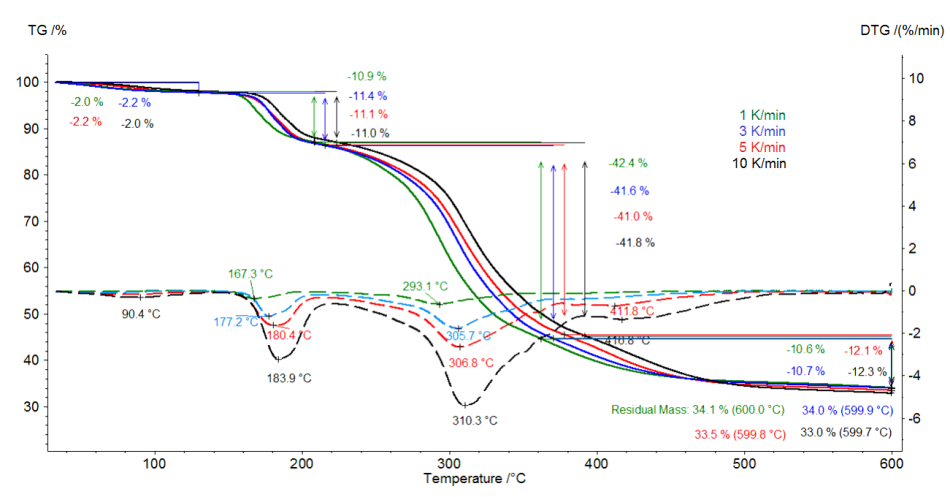Figure 2. TGA measurement on potassium clavulanate in pierced crucibles at different heating rates in a dynamic nitrogen atmosphere, solid lines: TGA, dashed lines: DTG.

2. TG measurements and Kinetics Neo

As the decomposition is dependent on heating rate, it allows for the evaluation of decomposition kinetics. NETZSCH Kinetics Neo software can be used for this. Kinetics Neo shows a kinetic model which has five consecutive steps of the nth order. This model calculates the kinetic parameters of each of the steps (e.g. pre-exponential factor and activation energy) however the water release is not taken into account for the calculation.

Figure 3 shows a comparison between the measured curves (dotted lines) and the calculated curves (solid lines) of the chosen 5 step model. It shows that the measured and calculated curves correlate very well.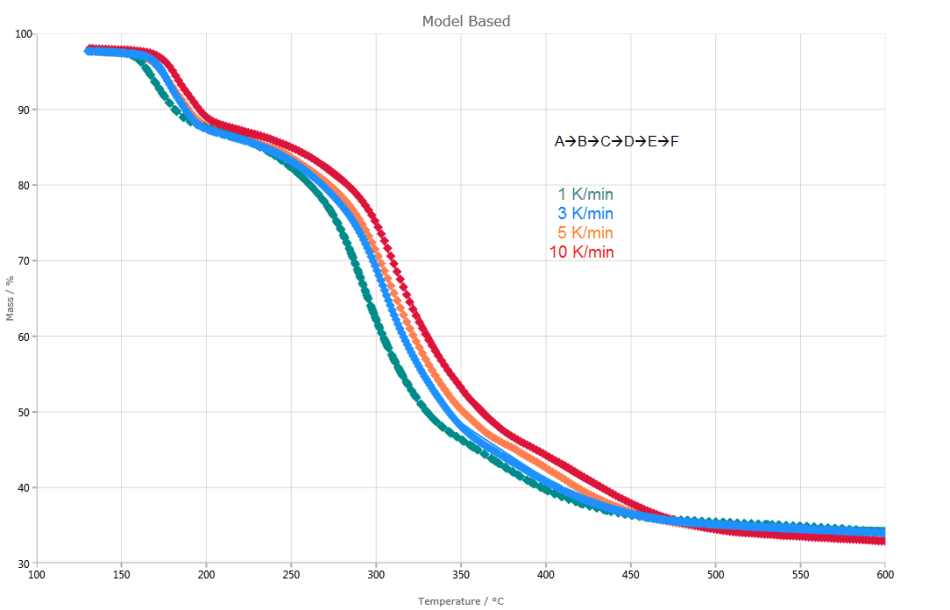Figure 3. Kinetic evaluation of the decomposition of potassium clavulanate. Dotted lines: measured curves; solid lines: calculated curves based on a five-step reaction of nth order. The correlation coefficient between measured and calculated curves amounts to >0.999.

3. Kinetics Neo can predict the behavior of the sample during storage at specified temperatures

Kinetics Neo will use the calculation to predict how the sample provided would behave during long time storage in varying temperature conditions. Figure 4 shows the 5 year forecast of the decomposition process of potassium clavulanate. This plot was produced in a few days by taking into account the measurements and evaluations of Kinetics Neo.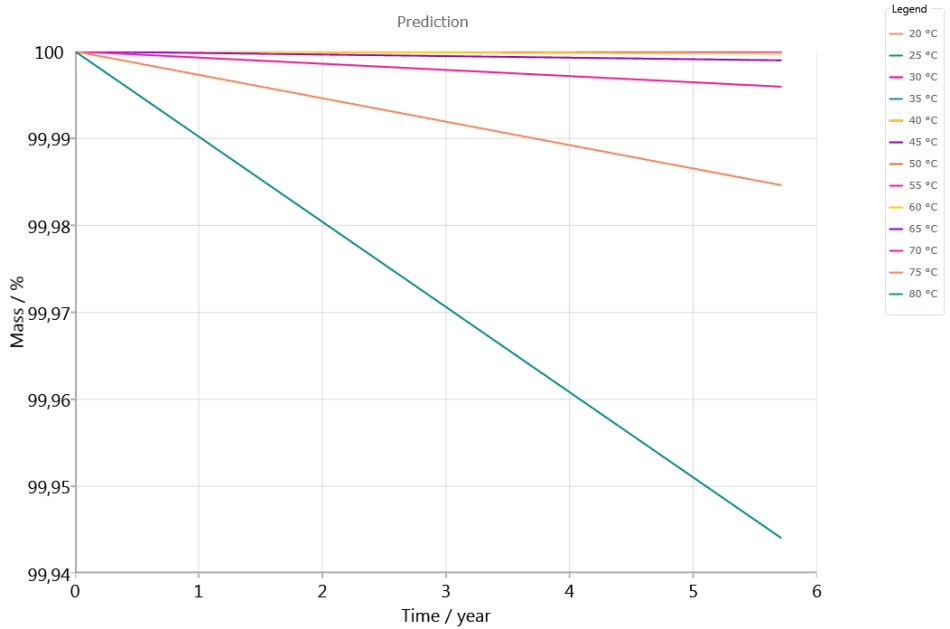Figure 4. Five-year forecast of the decomposition process of potassium clavulanate in a nitrogen atmosphere between 20 °C and 80 °C.

4. Validating the kinetics model ensures the accuracy of the calculation

The kinetic model that Kinetics Neo has calculated should be validated for the prediction of the decomposition under isothermal conditions. To do this, a potassium clavulanate sample (9.32 mg) was heated to 200 °C and then was kept isothermal for two hours.

In Figure 5 the mass losses are determined by comparing the measurements to those determined via prediction (Kinetics Neo).  This comparison shows that the two curves are congruent and therefore shows the reliability of the calculation.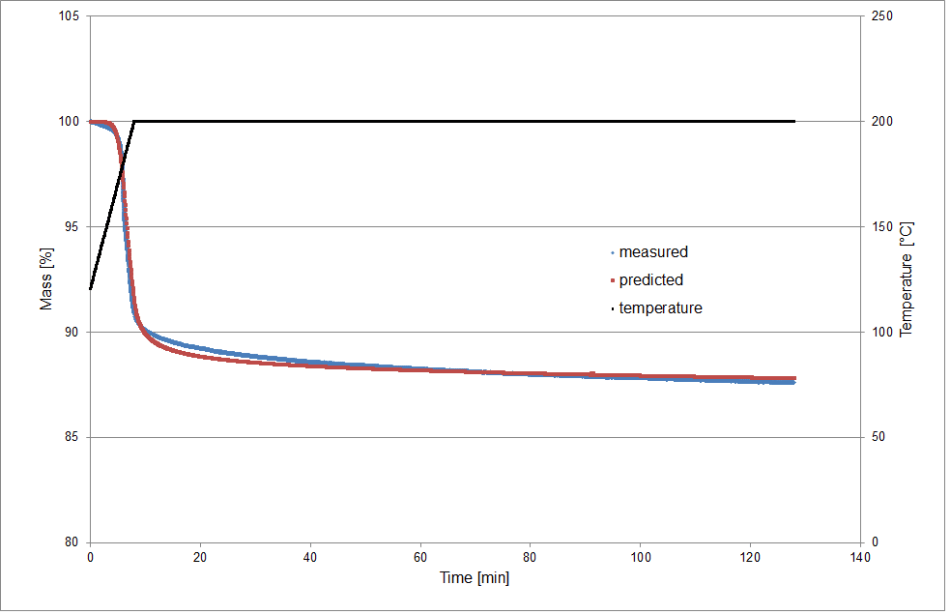Figure 5. Comparison of the measured and predicted mass change of potassium clavulanate during heating to 200 °C and isothermal segment; the release of surface water is not monitored.This information has been sourced, reviewed and adapted from materials provided by NETZSCH-Gerätebau GmbH.

## Citations

• APA

NETZSCH-Gerätebau GmbH. (2019, December 04). Reducing Testing Time of Pharmaceutical Drugs. AZoM. Retrieved on August 05, 2020 from https://www.azom.com/article.aspx?ArticleID=17342.

• MLA

NETZSCH-Gerätebau GmbH. "Reducing Testing Time of Pharmaceutical Drugs". AZoM. 05 August 2020. <https://www.azom.com/article.aspx?ArticleID=17342>.

• Chicago

NETZSCH-Gerätebau GmbH. "Reducing Testing Time of Pharmaceutical Drugs". AZoM. https://www.azom.com/article.aspx?ArticleID=17342. (accessed August 05, 2020).

• Harvard

NETZSCH-Gerätebau GmbH. 2019. Reducing Testing Time of Pharmaceutical Drugs. AZoM, viewed 05 August 2020, https://www.azom.com/article.aspx?ArticleID=17342.